# Map on collections in Swift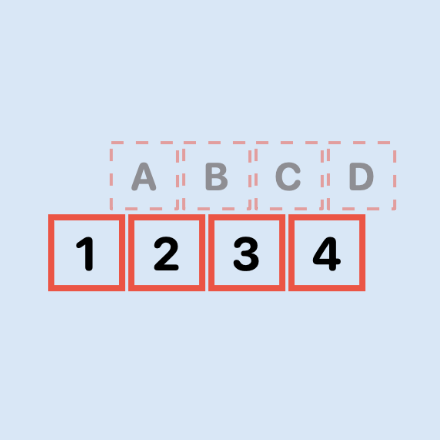Swift provides a number of map methods to transform elements in Swift collections and return the transformed data in a new Array. The transform is supplied in a trailing closure to the map function. This article looks at Map, FlatMap and CompactMap on collections in Swift as well as MapValues in Dictionary.

## Map on Array of Numbers and Strings

map is an instance method applied to a collection element such as an Array that applies some function/operation to each element in the collection and returns an array with the transformed elements. This operation to perform is passed in a trailing closure and can be any valid operation on the elements in the original collection. This example returns an array of the squares of the original numbers. Numbers can be converted to text or used in calculations and text case can be manipulated. The original collection remains unmodified by the map function.

 1let nums = [1, 2, 3, 4, 5]
2print(nums)
3print()
4
5let squared = nums.map { $0 *$0 }
6print(squared)
7print()
8
9let stringNums = nums.map { "\($0)" } 10print(stringNums) 11print() 12 13let numSquared = nums.map { "\($0) squared is \($0 *$0)" }
14print(numSquared)
15print()
16
17let strings = ["red", "orange", "yellow", "green", "blue", "indigo", "violet"]
18print(strings)
19let upperStrings = strings.map { $0.uppercased() } 20print(upperStrings)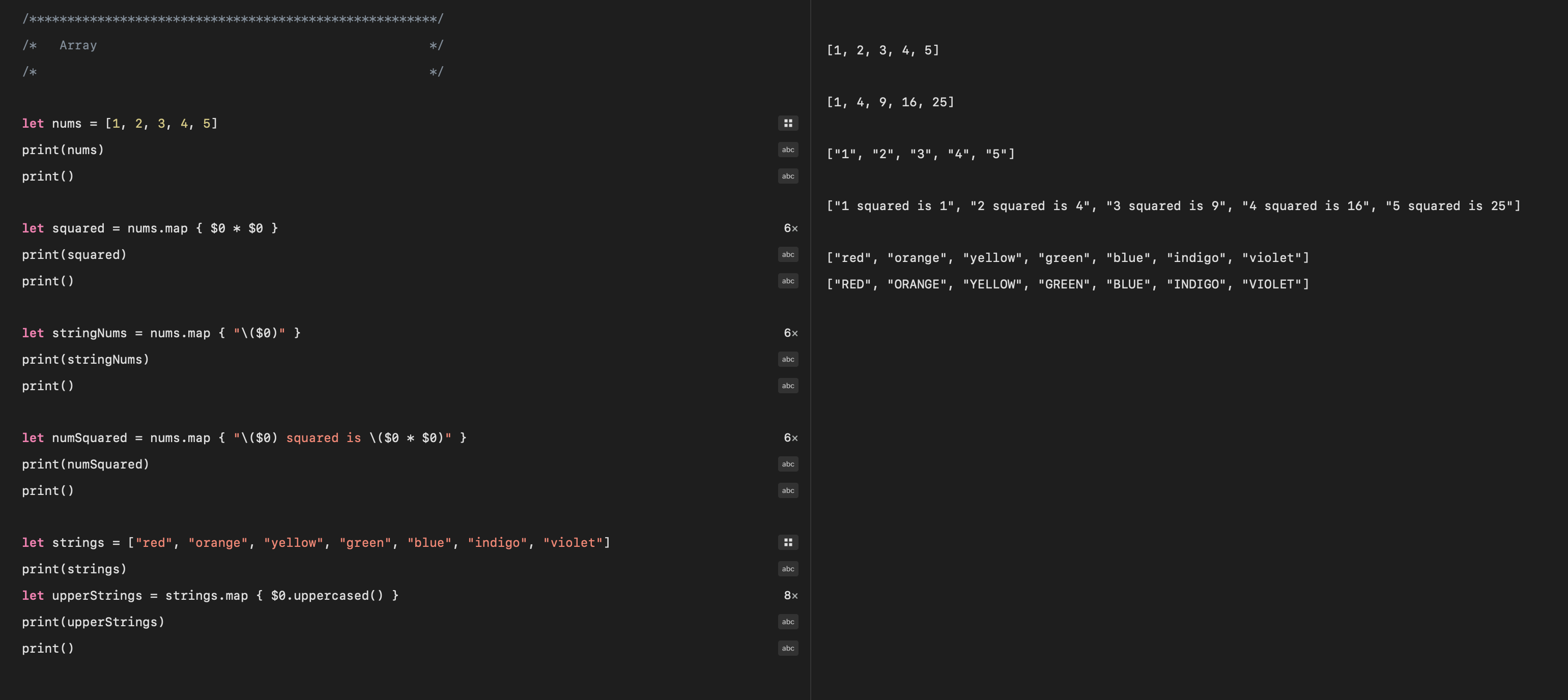Use Map to transform an array of numbers to another array with the specified closure ## Map on a Set Map can also be applied to Set, similar to Array. It returns an Array rather than a Set with elements created from applying the transform to the Set elements.  1let numSet = Set([1,2,3,2,3,4,5,6,-3,4,]) 2print("numSet = \(numSet)") 3print() 4 5let squaredSet = numSet.map {$0 * $0 } 6print(squaredSet) 7print("Type returned from map method on Set is \(type(of: squaredSet))") 8print() 9 10let sortedSquares = numSet.map {$0 * $0 }.sorted() 11print(sortedSquares) 12print()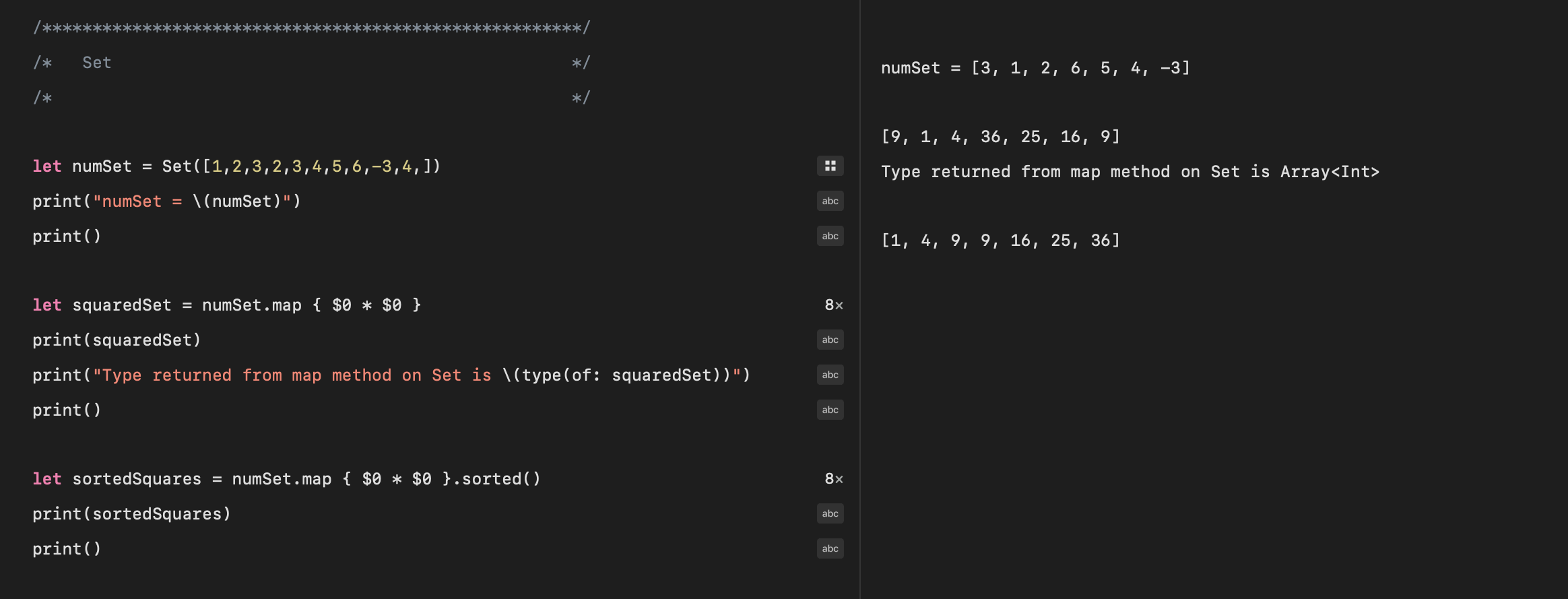Use Map to transform a Set of numbers returns an Array ## Map and mapValues on Dictionary Map on a Dictionary will allow the transform to work on the elements in the dictionary. Since the elements in the dictionary consist of a key/value pair, simply applying map to the dictionary will require accessing the key or value within the transform. The Map function will return an Array containing the transformed values. Swift provides mapValues that will return a new dictionary with the same keys as the original dictionary and the values transformed by the closure. The code shows that map returns an array of squared values and mapValues returns a new dictionary with the values squared for each key.  1/******************************************************/ 2/* Dictionary */ 3/* */ 4 5let dict = ["red": 1, 6 "orange": 2, 7 "yellow": 3, 8 "green": 4, 9 "blue": 5, 10 "indigo": 6, 11 "violet": 7] 12 13print(dict) 14print() 15 16let squareArray = dict.map {$0.value * $0.value } 17print(squareArray) 18print("Type returned from map method on Dictionary is \(type(of: squareArray))") 19print() 20 21let squareDict = dict.mapValues {$0 * $0 } 22print(squareDict) 23print("Type returned from mapValues method on Dictionary is \(type(of: squareDict))") 24print() 25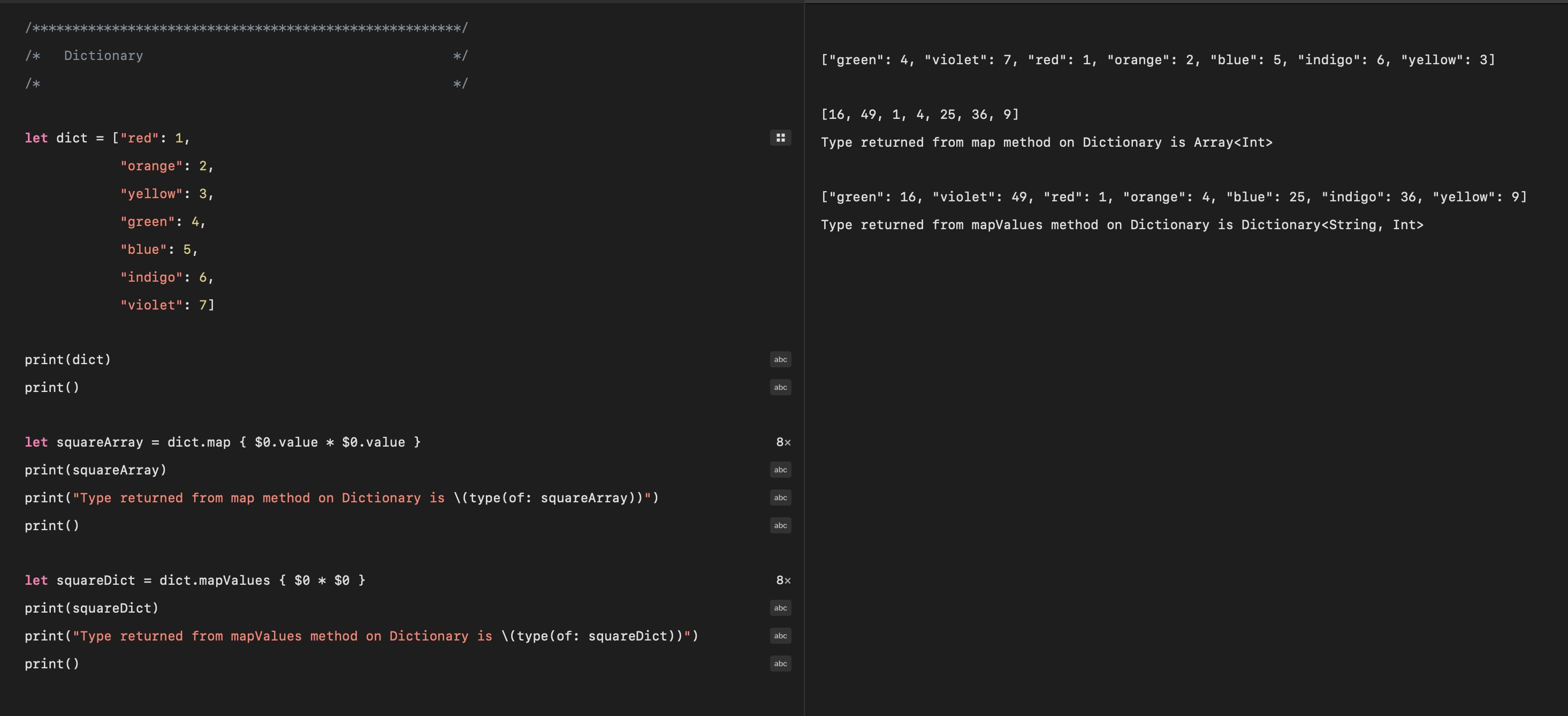Use Map to transform a Dictionary returns an Array ## FlatMap flatMap is used when the transform on the elements in the original collection results in a sequence for each element or when the original collection is a sequence of sequences. FlatMap can simply be used to concatenate a list of lists into a single list.  1let nums = [1, 2, 3, 4] 2print(nums) 3print() 4 5let expanded = nums.map { [$0, $0 *$0, $0 *$0 * $0] } 6print("expanded:\n\(expanded)") 7expanded.map { print($0) }
8print()
9
10let expanded2 = nums.flatMap { [$0,$0 * $0,$0 * $0 *$0] }
11print("expanded2:\n\(expanded2)")


In an Array of Arrays, FlatMap is used to convert to a single Array and then Map is used to transform the elements resulting in a new single Array with all the elements transformed.

 1let lists = [[1, 5, 3], [4, 1], [9, 2, 6],[5, 7, 2, 3]]
2print("\n\n\n\nList of lists")
3lists.map { print($0) } 4print() 5 6let flatList = lists.flatMap {$0 }
7print("flatList:\n\(flatList)")
8print()
9
10let flatSquares = lists
11    .flatMap { $0 } 12 .map {$0 * $0 } 13print("flatSquares:\n\(flatSquares)")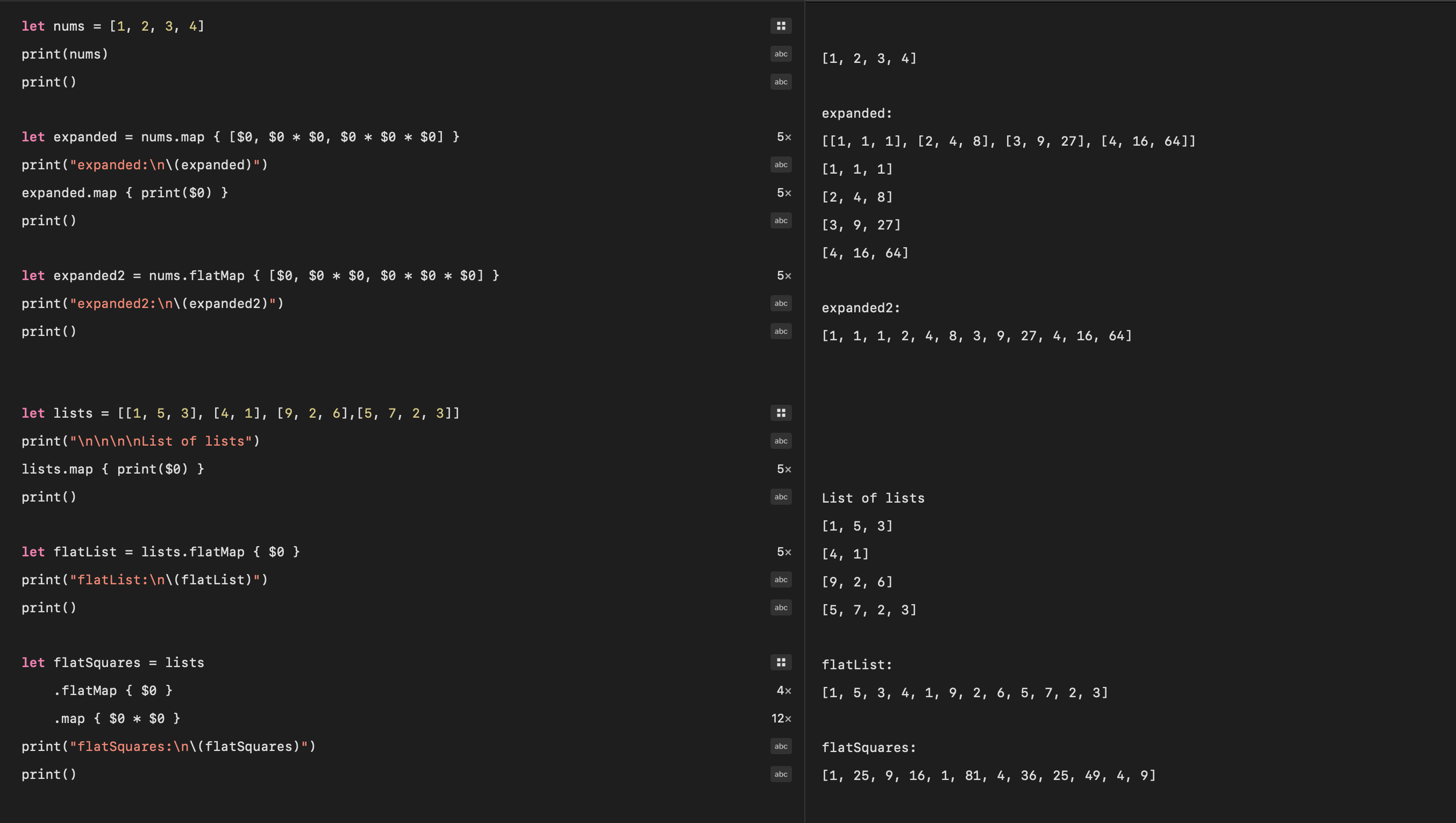Use FlatMap to collapse an array or arrays into a single Array ## CompactMap compactMap is used to return an array of non-nil values when the transform can result in nil values for elements in the sequence. Useful when the transform produces an array of optional values. This code finds words starting with uppercase letters in the string. The map function will return the option values with some of the elements being nil, whereas the compactMap just returns the non-nil values and unwraps the optionals.  1import Foundation 2 3let text = "The Rain in Spain falls mainly in the Plain" 4let words = text.components(separatedBy: .whitespacesAndNewlines) 5print(words) 6print() 7 8let cap1 = words.map {$0.first?.isUppercase ?? false ? $0 : nil } 9print(cap1) 10print() 11 12let cap2 = words.compactMap {$0.first?.isUppercase ?? false ? \$0 : nil }
13print(cap2)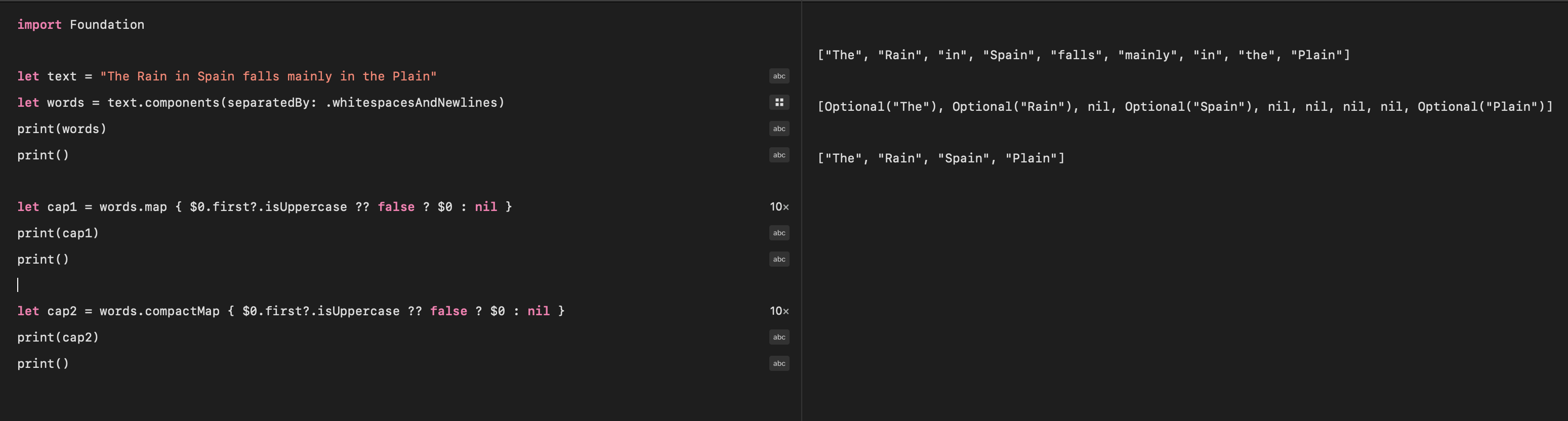Use CompactMap to eliminate nil values from the transformed Array

## Conclusion

There is frequently the need to transform one collection into another and while this can be done with a For or ForEach loop, Swift provides Map functions tailored to this requirement. The map function take a trailing closure with code to apply to each element in the collection and return the transformed array. All map functions return an Array regardless of the type of the collection to which the map is applied. The Dictionary type also provides mapValues that returns a dictionary with the original keys and the values transformed. FlatMap function is used to collapse (Flatten) a sequence of sequences into a single sequence, while CompactMap function is used to eliminate nil values from the transformed collection.